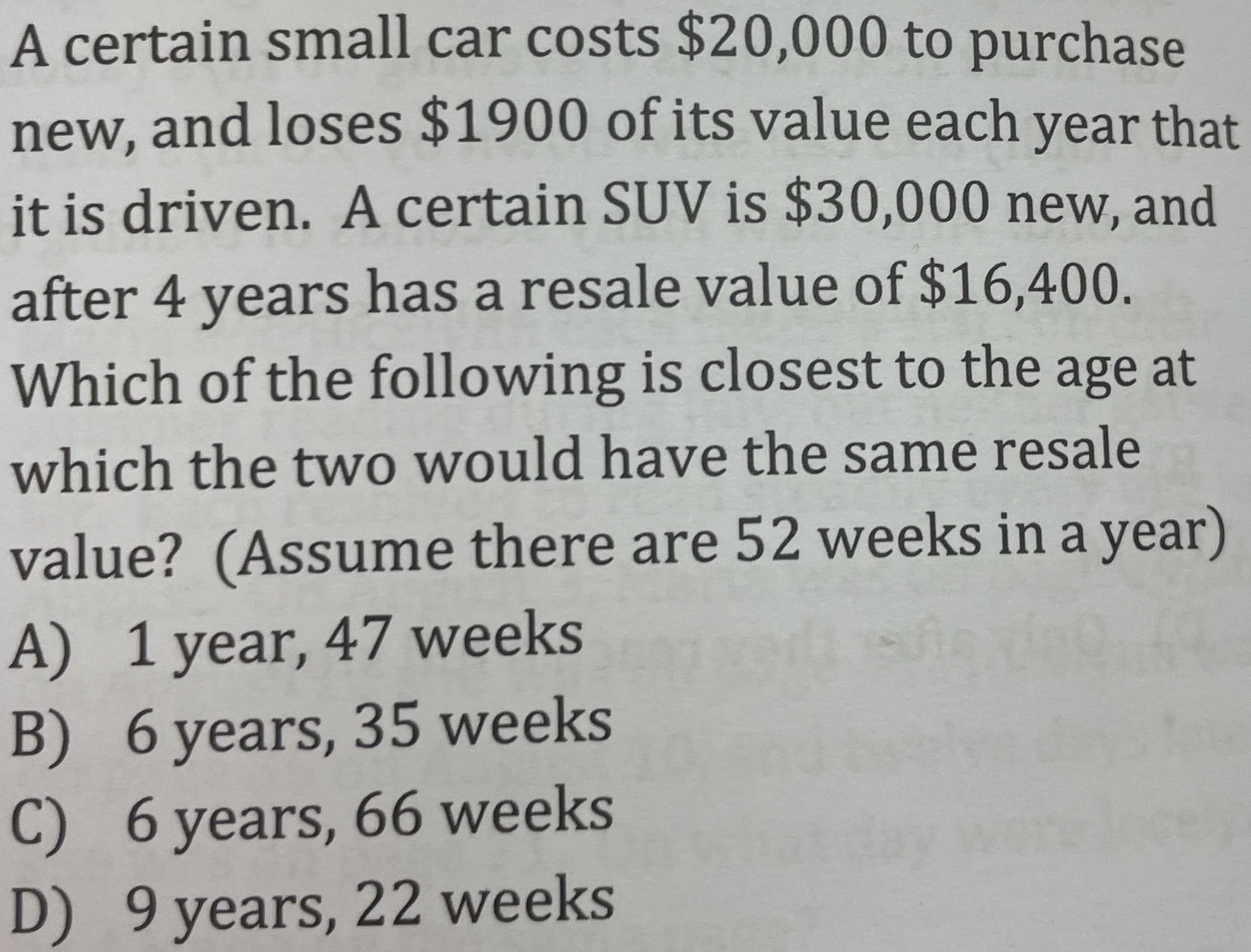### ¿Todavía tienes preguntas de matemáticas?

Pregunte a nuestros tutores expertos
Algebra
PreguntaA certain small car costs $$\ 20,000$$ to purchase new, and loses $$\ 1900$$ of its value each year that it is driven. A certain SUV is $$\ 30,000$$ new, and after $$4$$ years has a resale value of $$\ 16,400$$ . Which of the following is closest to the age at which the two would have the same resale value? (Assume there are $$52$$ weeks in a year) A) $$1$$ year, $$47$$ weeks B) $$6$$ years, $$35$$ weeks C) $$6$$ years, $$66$$ weeks D) $$9$$ years, $$22$$ weeks

$$x = 6 \text{ years } , 35 \text{ weeks }$$